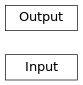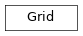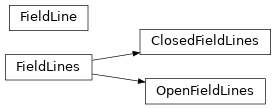API reference

pfsspy Package

Functions

 pfss(input) Compute PFSS model.

Classes

 Input(br, nr, rss) Input to PFSS modelling. Output(alr, als, alp, grid[, input_map]) Output of PFSS modelling.

Class Inheritance Diagrampfsspy.grid Module

Classes

 Grid(ns, nphi, nr, rss) Grid on which the pfsspy solution is calculated.

Class Inheritance Diagrampfsspy.fieldline Module

Classes

 ClosedFieldLines(field_lines) A set of closed field lines. FieldLine(x, y, z, output) A single magnetic field line. FieldLines(field_lines) A collection of FieldLine. OpenFieldLines(field_lines) A set of open field lines.

Class Inheritance Diagrampfsspy.tracing Module

Classes

 FortranTracer([max_steps, step_size]) Tracer using Fortran code. PythonTracer([atol, rtol]) Tracer using native python code. Tracer() Abstract base class for a streamline tracer.

Class Inheritance Diagrampfsspy.utils Module

Functions

 car_to_cea(m[, method]) Reproject a plate-carée map in to a cylindrical-equal-area map. carr_cea_wcs_header(dtime, shape) Create a Carrington WCS header for a Cylindrical Equal Area (CEA) projection. fix_hmi_meta(hmi_map) Fix non-compliant FITS metadata in HMI maps. is_car_map(m[, error]) Returns True if m is in a plate carée projeciton. is_cea_map(m[, error]) Returns True if m is in a cylindrical equal area projeciton. is_full_sun_synoptic_map(m[, error]) Returns True if m is a synoptic map spanning the solar surface. load_adapt(adapt_path) Parse adapt .fts file as a sunpy.map.MapSequence roll_map(m[, lh_edge_lon, method]) Roll an input synoptic map so that it's left edge corresponds to a specific Carrington longitude.

pfsspy.analytic Module

Analytic inputs and solutions to the PFSS equations.

This sub-module contains functions to generate solutions to the PFSS equations in the case where the input field is a single spherical harmonic, specified with the spherical harmonic numbers l, m.

All angular quantities must be passed as astropy quantities. All radial quantities are passed normalised to the source surface radius, and therefore can be passed as normal scalar values.

Angular definitions

• theta is the polar angle, in the range $$0, \pi$$ (ie. the co-latitude).

• phi is the azimuthal angle, in the range $$0, 2\pi$$.

Using this module requires sympy to be installed.

Functions

 Bphi(l, m, zss) Analytic phi component of magnetic field on the source surface. Br(l, m, zss) Analytic radial component of magnetic field on the source surface. Btheta(l, m, zss) Analytic theta component of magnetic field on the source surface.